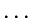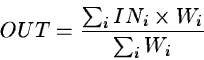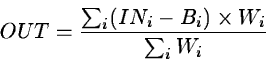Next: Cleaning Images Up: CCD Detectors Previous: Bias Corrections

## Averaging and Merging Frames

Three different techniques for averaging have been introduced. The first is a straight forward simple average without regard for statistical weights or possible non-statistical pixel values. The second average takes account of the fact that the images being averaged may have different statistical weights. The difference in statistical weights may arise, since one image may have been exposed through clouds or may have different noise characteristics for some reason or other. The third averaging technique not only takes account of possible different statistical weights and mean values, but also excludes from the resulting average those pixels which differ from the others in a non-statistical way.

The average functions mentioned above will normally produce a result frame whose world coordinates are the intersection of the father frames' world coordinates. It is possible to specify that the result be the union of the world coordinates of the father frames. In this case, the average functions will produce merged images.

Simple averaging -- This function will take the given frames and produce a simple average of the pixel values. The inputs are either a list of frames names separated by commas, or a catalog reference. In the latter case, all frames in the catalog will be averaged to produce the result. The command is:

AVERAGE/IMAGE OUT = IN_1,IN_2,,IN_n [M] [null]
AVERAGE/IMAGE OUT = catalog.CAT [M] [null]
where in the first case, the series of specific input frames will be averaged and in the second case all entries in the specified catalog will be averaged to produce the output frame. The optional parameter M determines whether or not the images will be merged in world coordinate space. The optional parameter null is used to specify the value that will be used to replace invalid pixels.

Weighted averaging -- This function is very similar to the simple average function only each input frame must have a precalculated weighting factor. The average is calculated according to the following formula:(18.10)

The function is called as:

AVERAGE/WEIGHT OUT = IN_1,IN_2,,IN_n [M] [null]
AVERAGE/WEIGHT OUT = catalog.CAT [M] [null]
where the parameters have exactly the same meaning as in the simple average command. The weighting factor has to be calculated beforehand with the command COMPUTE/WEIGHT or has to be entered by hand in a real descriptor WEIGHT.

Averaging statistically consistent values --  This command differs somewhat from the purely mathematical functions of the previous two commands in the sense that it tries to anticipate some of the astronomical content of the input data frames. The function requires that four auxiliary parameters (in descriptors) be defined for each input frame before it can process the frames. These parameters can be defined by the function WRITE/DESCRIPTOR and are:

• LHCUTS(5),LHCUTS(6) -- low and high limits for valid pixels
• O_TIME(7) -- exposure time
• FLAT_BCK(1) -- mean background value.
The function calculates the following:(18.11)

with the restriction that only those pixels satisfying the condition(18.12)

are accepted into the average. The threshold for rejection of pixels is a selectable parameter in the command. The default is shown.

The function is referenced to as:

AVERAGE/WINDOW OUT = IN_1,IN_2,,IN_n bgerr,snoise
AVERAGE/WINDOW OUT = catalog.CAT bgerr,snoise

Merging frames --  Often in order to properly merge frames, it is necessary to adjust the world coordinates of the frames so that the individual objects in each frame have the same world coordinates in the various different frames. This then allows the averaging routines to proceed to combine the frames correctly. Notice that almost all the MIDAS routines operate in world coordinates.Next: Cleaning Images Up: CCD Detectors Previous: Bias Corrections
Petra Nass
1999-06-15